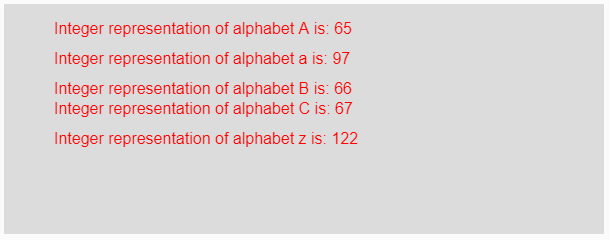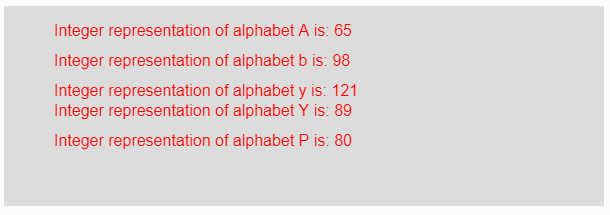# p5.js | unchar() function

The unchar() function in p5.js is used to convert a single-character string into its corresponding integer representation. This function is just opposite to the char() function.

Syntax:

`unchar(value)`

Parameters: This function accepts single parameter value which is to be converted into its corresponding integer representation. This value will be any single-character string or an array of single-characters.

Return Value: It returns the converted integer representation of single-character string.

Below program illustrates the unchar() function in p5.js:

Example 1: This example uses unchar() function to converts a single-character string into its corresponding integer representation.

 `function` `setup() {  ` `  `  `    ``// Creating Canvas size ` `    ``createCanvas(600, 230);  ` `}  ` `  `  `function` `draw() {  ` `      `  `    ``// Set the background color  ` `    ``background(220);  ` `    `  `    ``// Initializing some values ` `    ``let Value1 = ``"A"``; ` `    ``let Value2 = ``"a"``; ` `    ``let Value3 = ``"B"``; ` `    ``let Value4 = ``"C"``;  ` `    ``let Value5 = ``"z"``; ` `    `  `    ``// Calling to unchar() function. ` `    ``let A = unchar(Value1); ` `    ``let B = unchar(Value2); ` `    ``let C = unchar(Value3); ` `    ``let D = unchar(Value4); ` `    ``let E = unchar(Value5); ` `    `  `    ``// Set the size of text  ` `    ``textSize(16);  ` `      `  `    ``// Set the text color  ` `    ``fill(color(``'red'``));  ` `    `  `    ``// Getting integer representation ` `    ``text(``"Integer representation of alphabet A is: "` `+ A, 50, 30); ` `    ``text(``"Integer representation of alphabet a is: "` `+ B, 50, 60); ` `    ``text(``"Integer representation of alphabet B is: "` `+ C, 50, 90); ` `    ``text(``"Integer representation of alphabet C is: "` `+ D, 50, 110); ` `    ``text(``"Integer representation of alphabet z is: "` `+ E, 50, 140); ` `}  `

Output:Example 2: This example uses unchar() function to converts a single-character string into its corresponding integer representation.

 `function` `setup() {  ` `  `  `    ``// Creating Canvas size ` `    ``createCanvas(600, 200);  ` `}  ` `  `  `function` `draw() {  ` `      `  `    ``// Set the background color  ` `    ``background(220);  ` `    `  `    ``// Calling to unchar() function. ` `    ``let A = unchar(``"A"``); ` `    ``let B = unchar(``"b"``); ` `    ``let C = unchar(``"y"``); ` `    ``let D = unchar(``"Y"``); ` `    ``let E = unchar(``"P"``); ` `    `  `    ``// Set the size of text  ` `    ``textSize(16);  ` `      `  `    ``// Set the text color  ` `    ``fill(color(``'red'``));  ` `    `  `    ``// Getting integer representation ` `    ``text(``"Integer representation of alphabet A is: "` `+ A, 50, 30); ` `    ``text(``"Integer representation of alphabet b is: "` `+ B, 50, 60); ` `    ``text(``"Integer representation of alphabet y is: "` `+ C, 50, 90); ` `    ``text(``"Integer representation of alphabet Y is: "` `+ D, 50, 110); ` `    ``text(``"Integer representation of alphabet P is: "` `+ E, 50, 140); ` `}     `

Output:Reference: https://p5js.org/reference/#/p5/unchar

My Personal Notes arrow_drop_upCheck out this Author's contributed articles.

If you like GeeksforGeeks and would like to contribute, you can also write an article using contribute.geeksforgeeks.org or mail your article to contribute@geeksforgeeks.org. See your article appearing on the GeeksforGeeks main page and help other Geeks.

Please Improve this article if you find anything incorrect by clicking on the "Improve Article" button below.

Article Tags :

Be the First to upvote.

Please write to us at contribute@geeksforgeeks.org to report any issue with the above content.